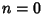## Indicial Equation

The Recurrence Relation obtained during application of the Frobenius Method of solving a second-order ordinary differential equation. The indicial equation (also called the Characteristic Equation) is obtained by noting that, by definition, the lowest order term(that corresponding to) must have a Coefficient of zero. For an example of the construction of an indicial equation, see Bessel Differential Equation.

1. If the two Roots are equal, only one solution can be obtained.

2. If the two Roots differ by a noninteger, two solutions can be obtained.

3. If the two Roots differ by an Integer, the larger will yield a solution. The smaller may or may not.

References

Morse, P. M. and Feshbach, H. Methods of Theoretical Physics, Part I. New York: McGraw-Hill, pp. 532-534, 1953.